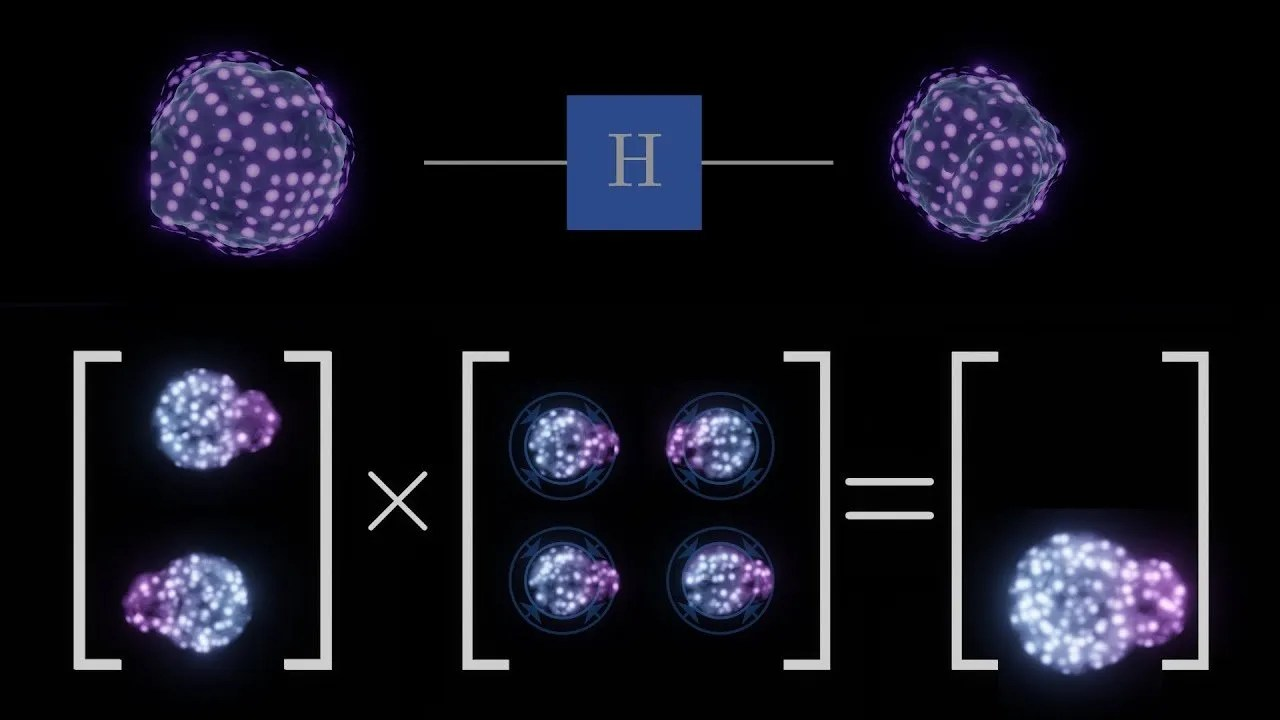# Just How Do Quantum Computers Work?

• IntroductionFascinating video explaining quantum computers beyond just “qubits can be 0 and 1 at the same time!”

Time stamps:

• 0:00 Introduction
• 0:32 Complex Numbers
• 2:20 Qubits
• 4:26 Single-Qubit Gates
• 7:09 Entanglement
• 8:30 Observation Is Entanglement
• 9:45 Permutations
• 10:00 Multi-Qubit Gates
• 10:20 Entanglement With Single-Qubit Gates
• 11:28 Recreating Classical Logic Gates
• 12:36 A Quantum Multiplier
• 13:45 The Oracle Of Grover’s Algorithm
• 15:45 The Rest Of Grover’s Algorithm
• 18:28 The Effectiveness Of Grover’s Algorithm